# Selina Solutions Concise Mathematics Class 6 Chapter 1: Number System

Selina Solutions Concise Mathematics Chapter 1 Number System with accurate answers provided here for Class 6 students. The various operations on the Number System mentioned in this Chapter are explained in brief. Students who find difficulty in solving the textbook questions are suggested to go through these Selina Solutions. These Solutions play a vital role in helping students, who aspire to score good marks in the academic field. Practising Selina Solutions Concise Mathematics Class 6 repeatedly will boost students’ confidence in facing the examination.

The Number System is based on Consolidating the sense of numberness, i.e. uniting or combining the numbers. Estimating numbers, Natural numbers and Whole numbers, International system, Comparing Whole numbers are some topics covered in this Chapter. The problems help students to solve tricky questions in an easy way. Students can download the solutions in the PDF format from the links given below.

## Selina Solutions Concise Mathematics Class 6 Chapter 1 Number System Download PDF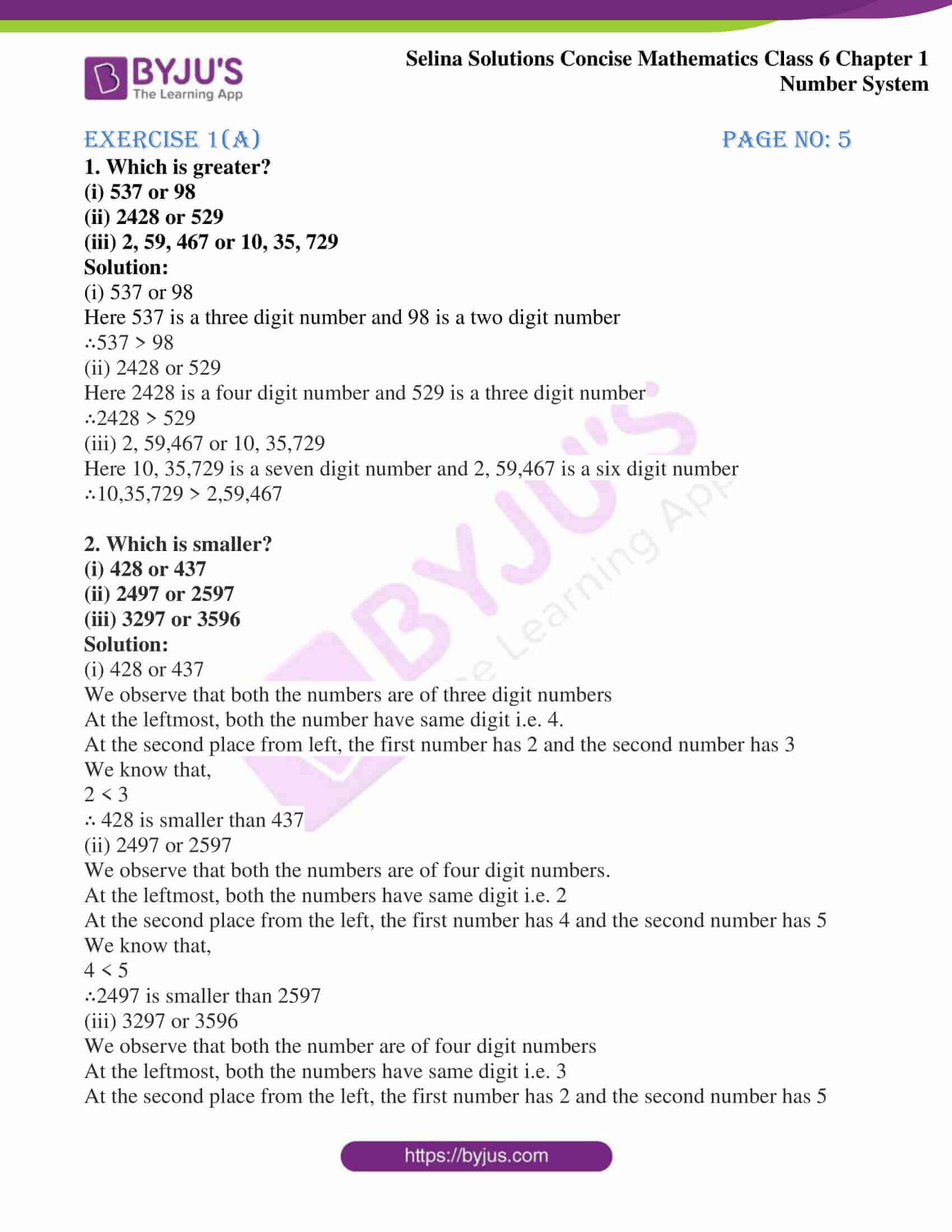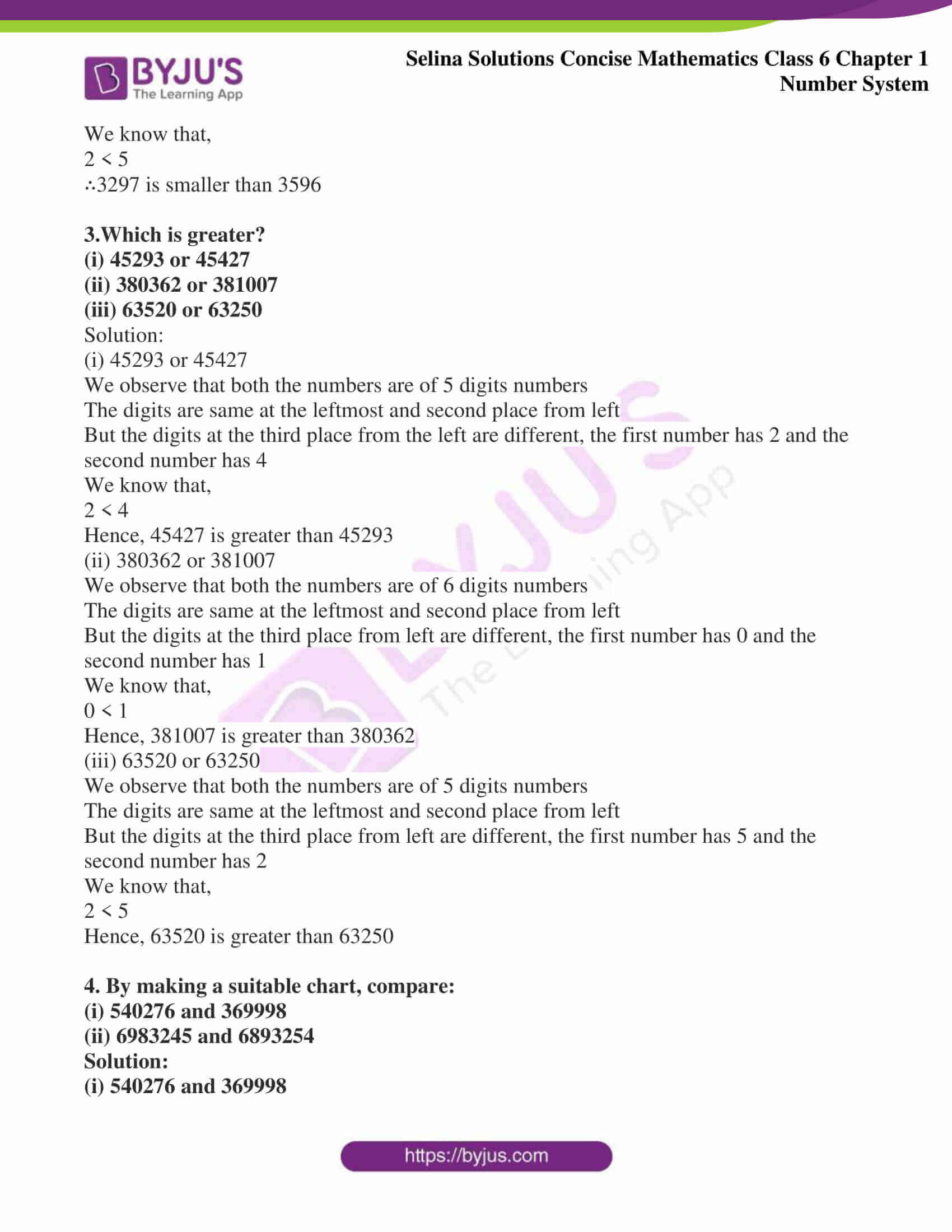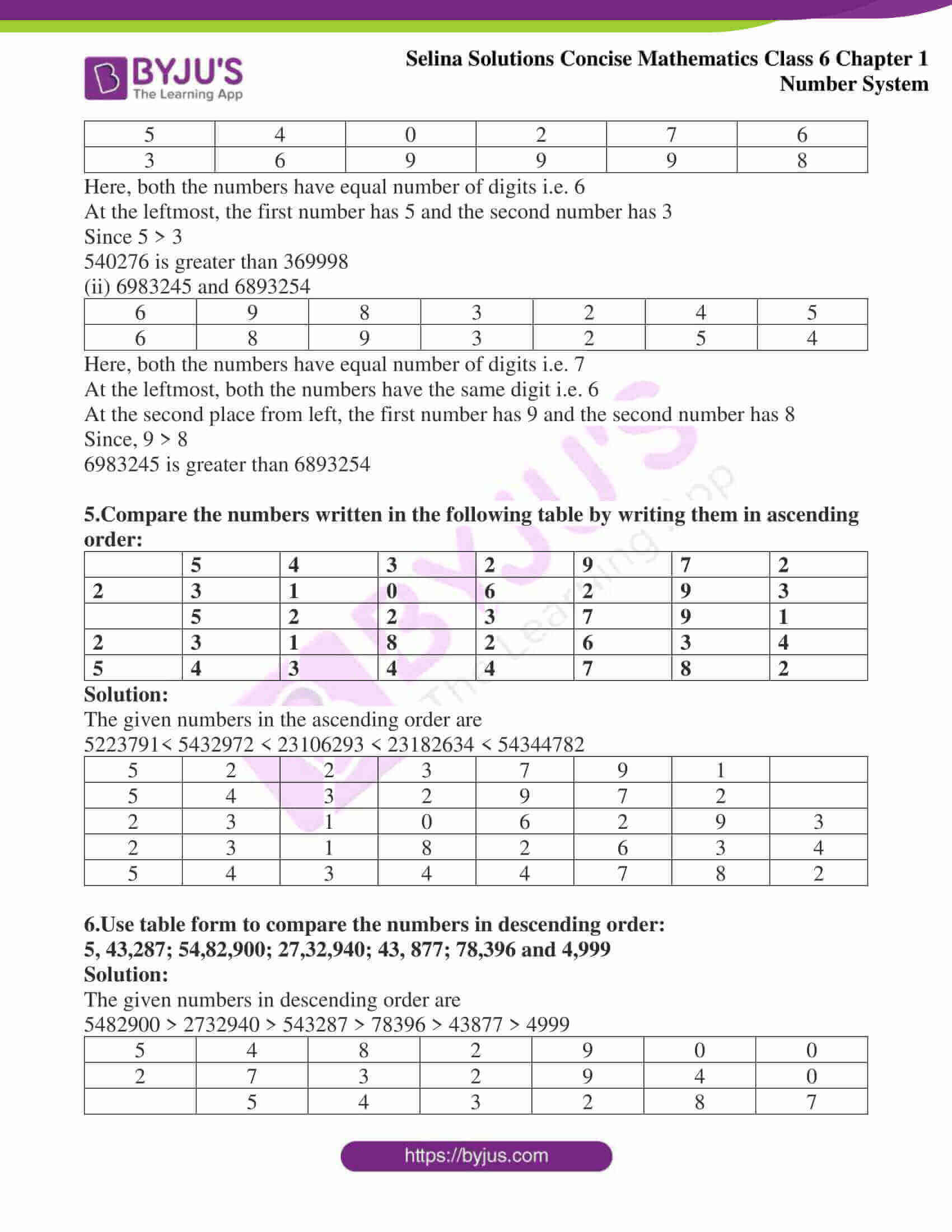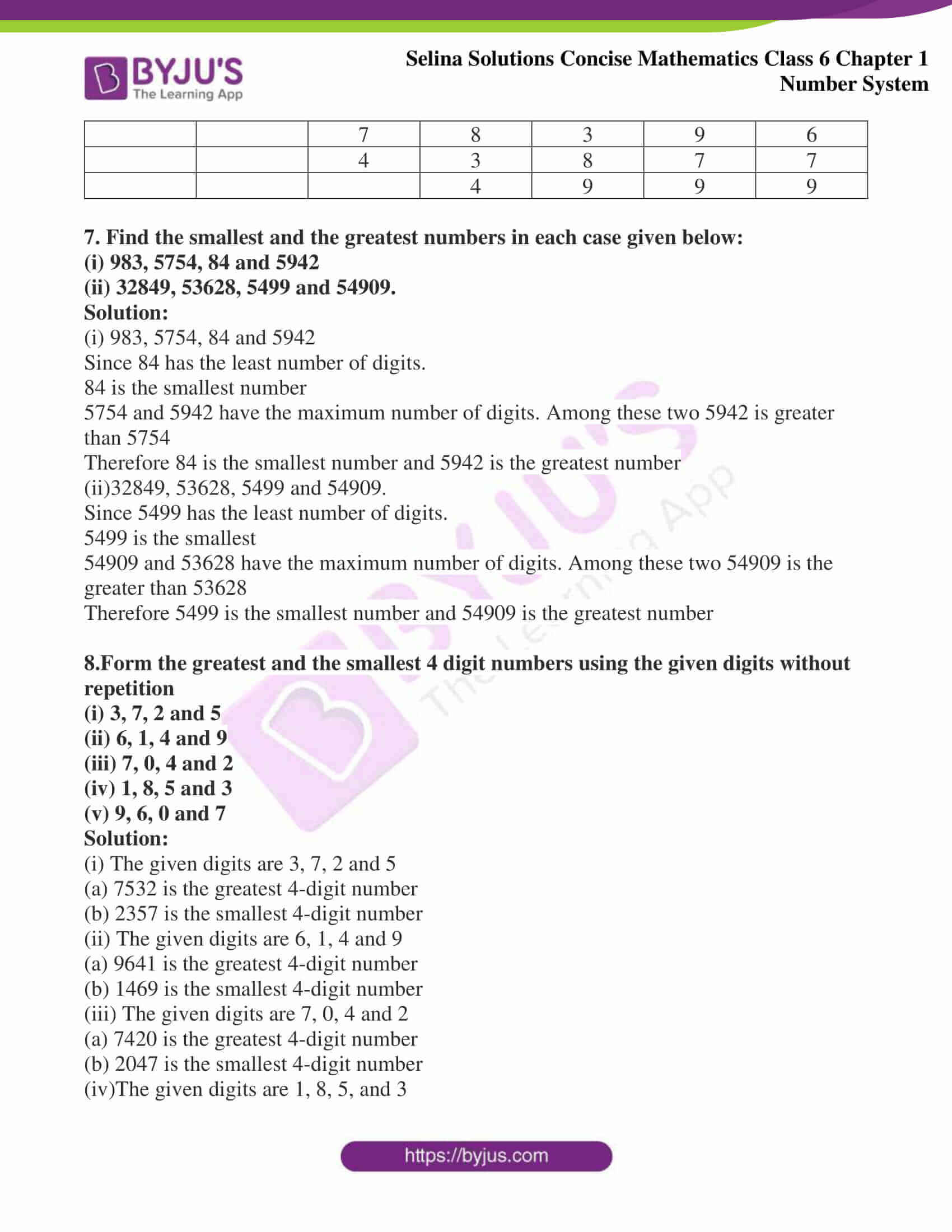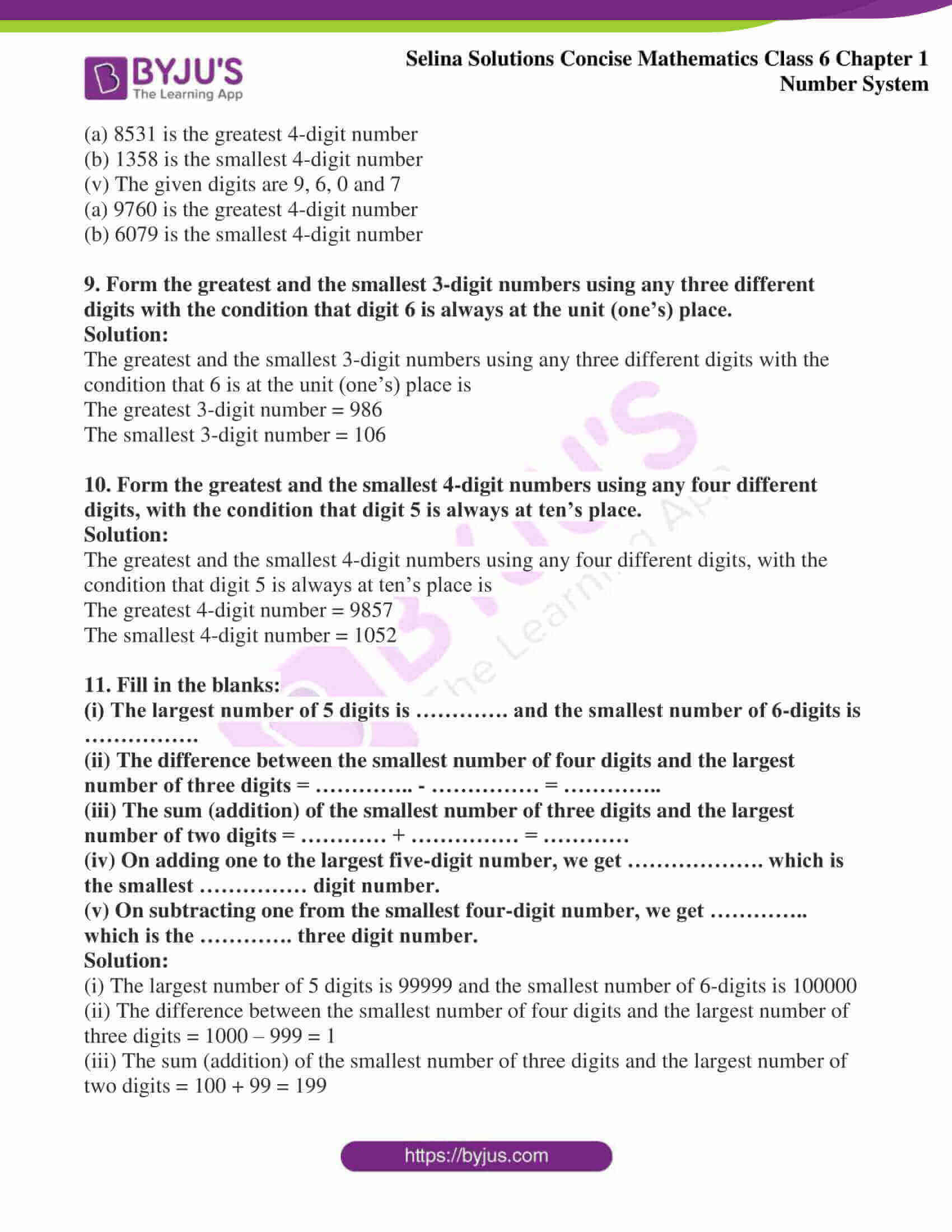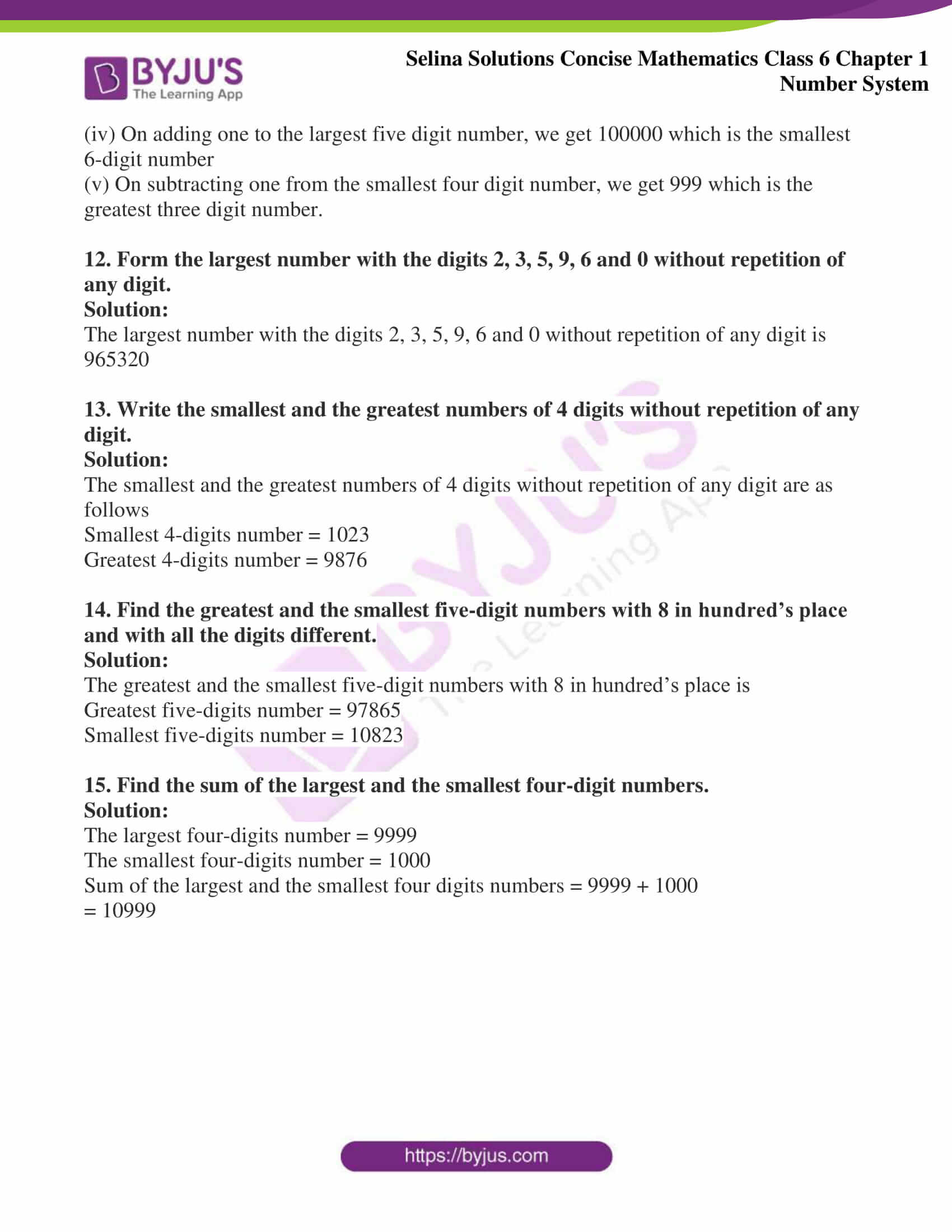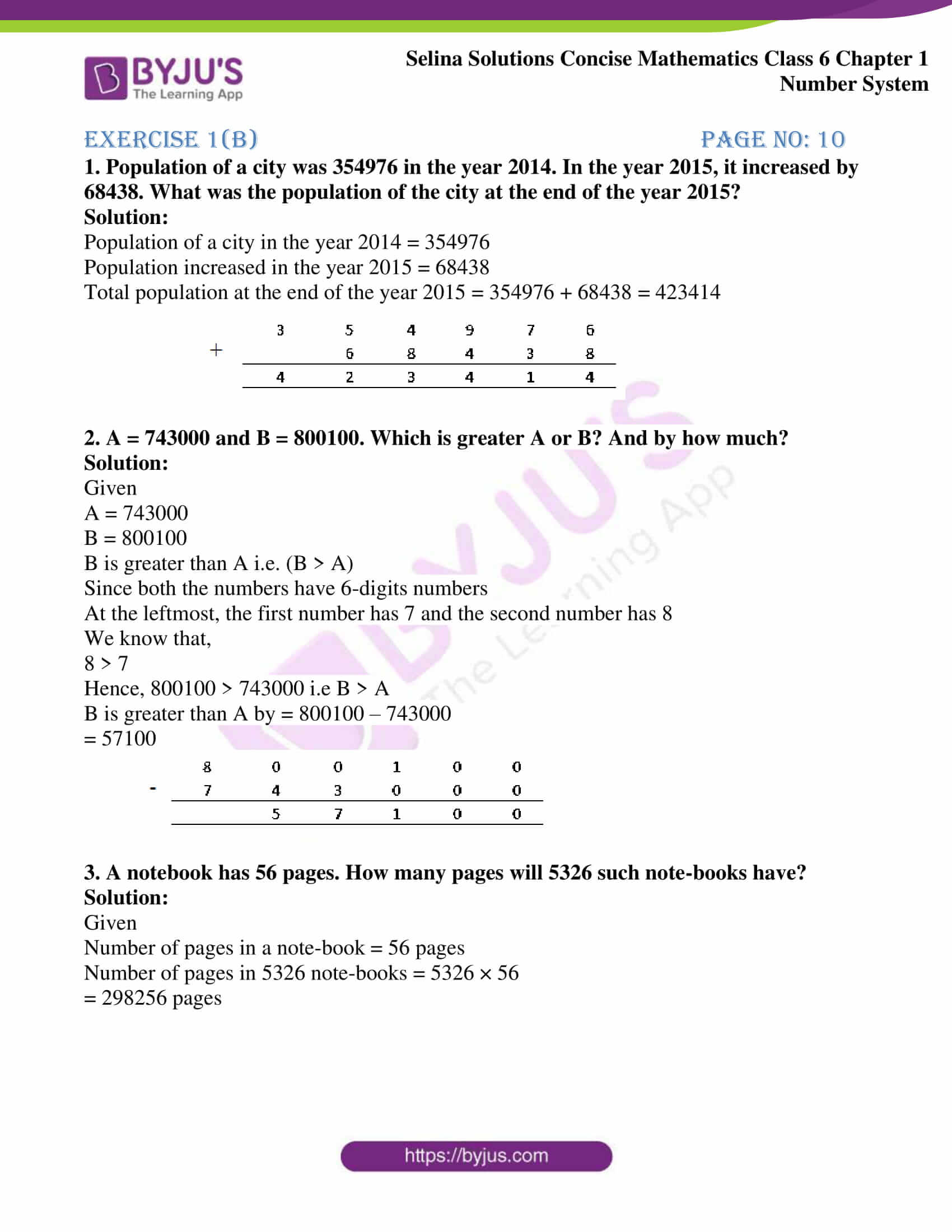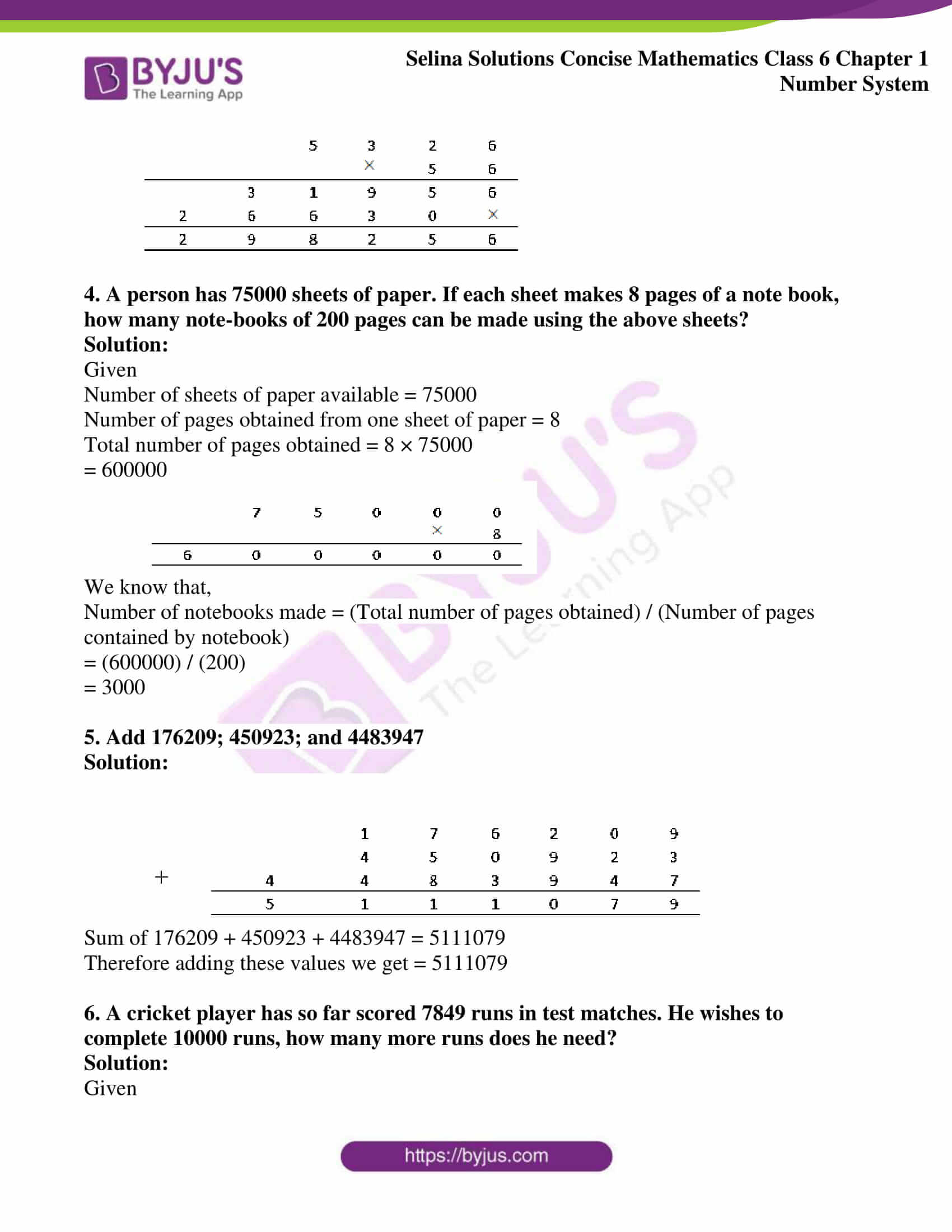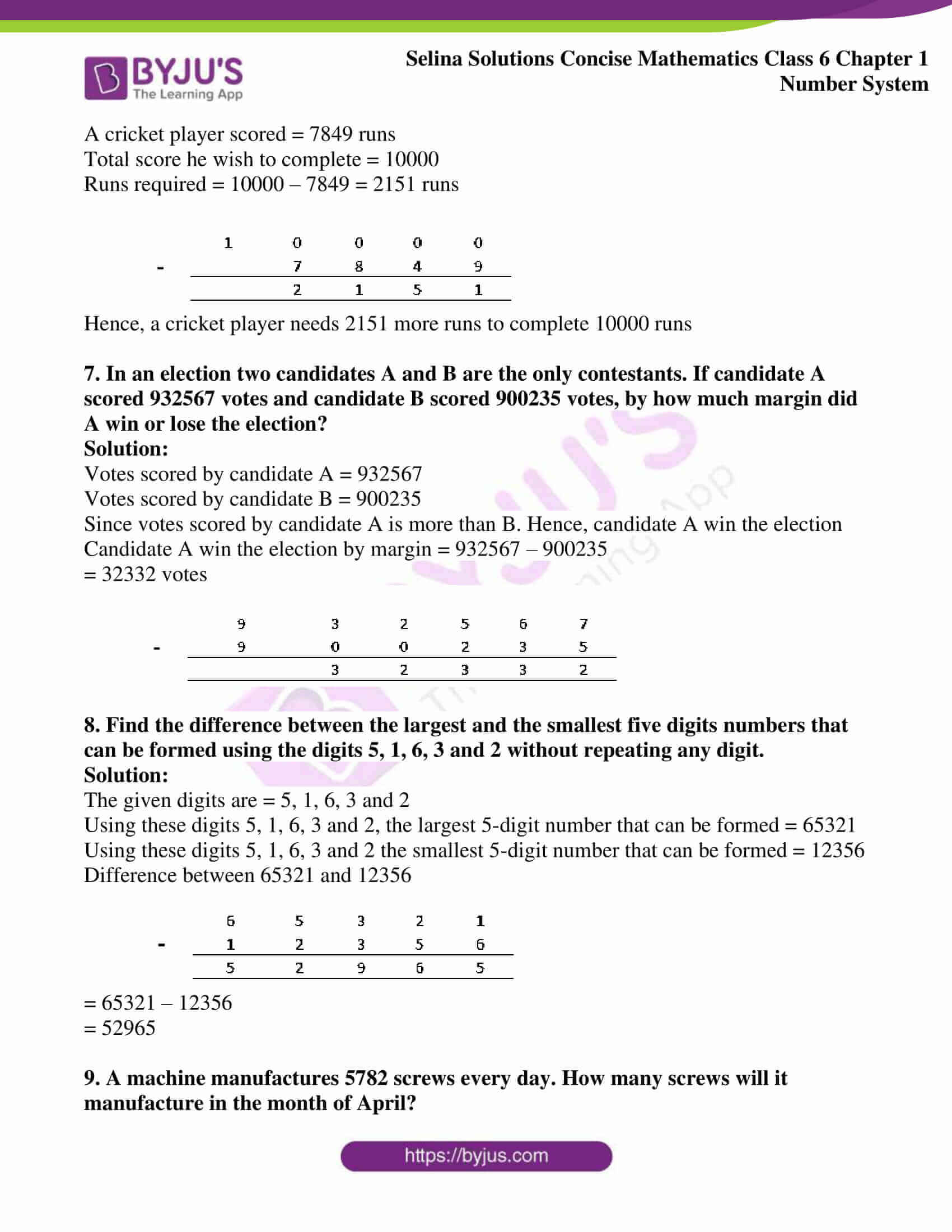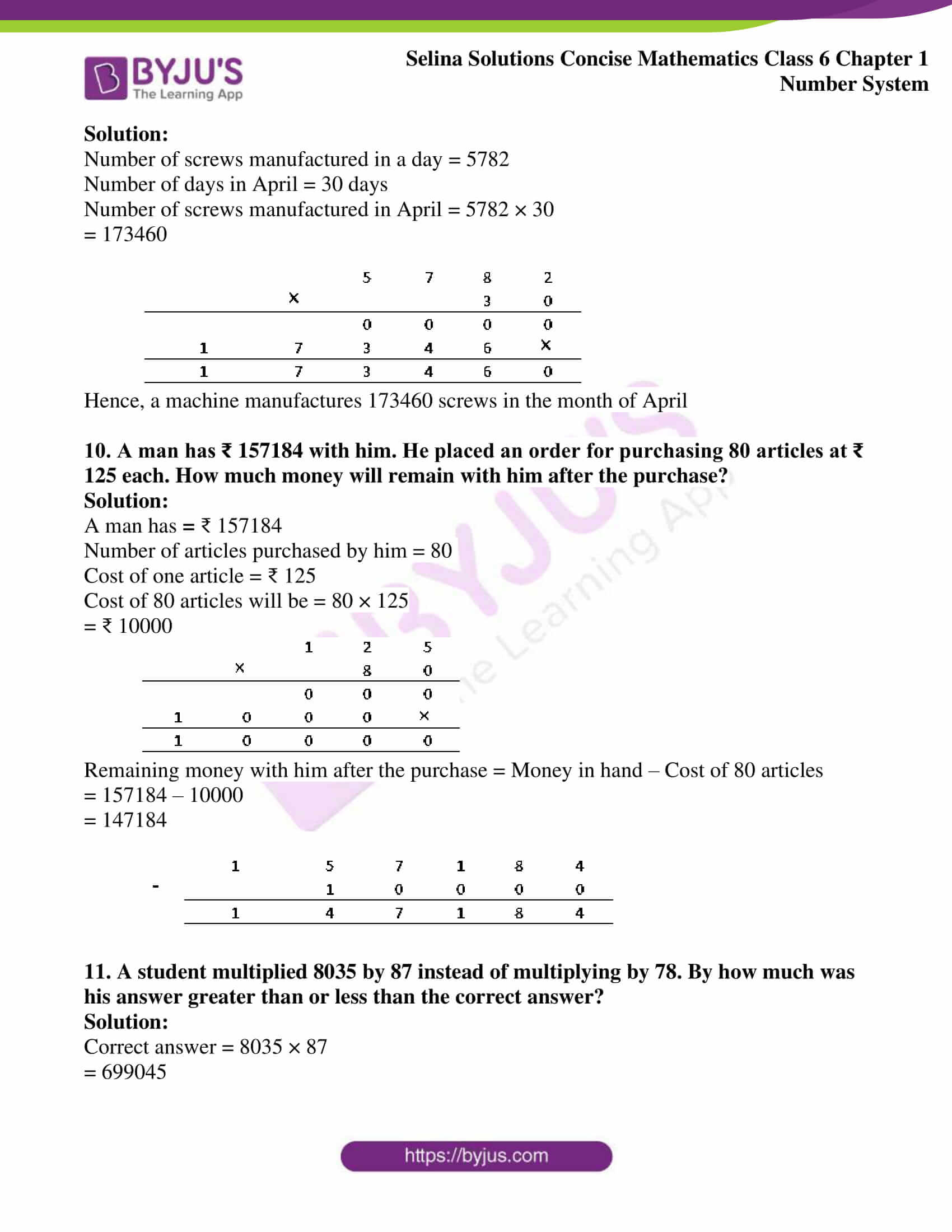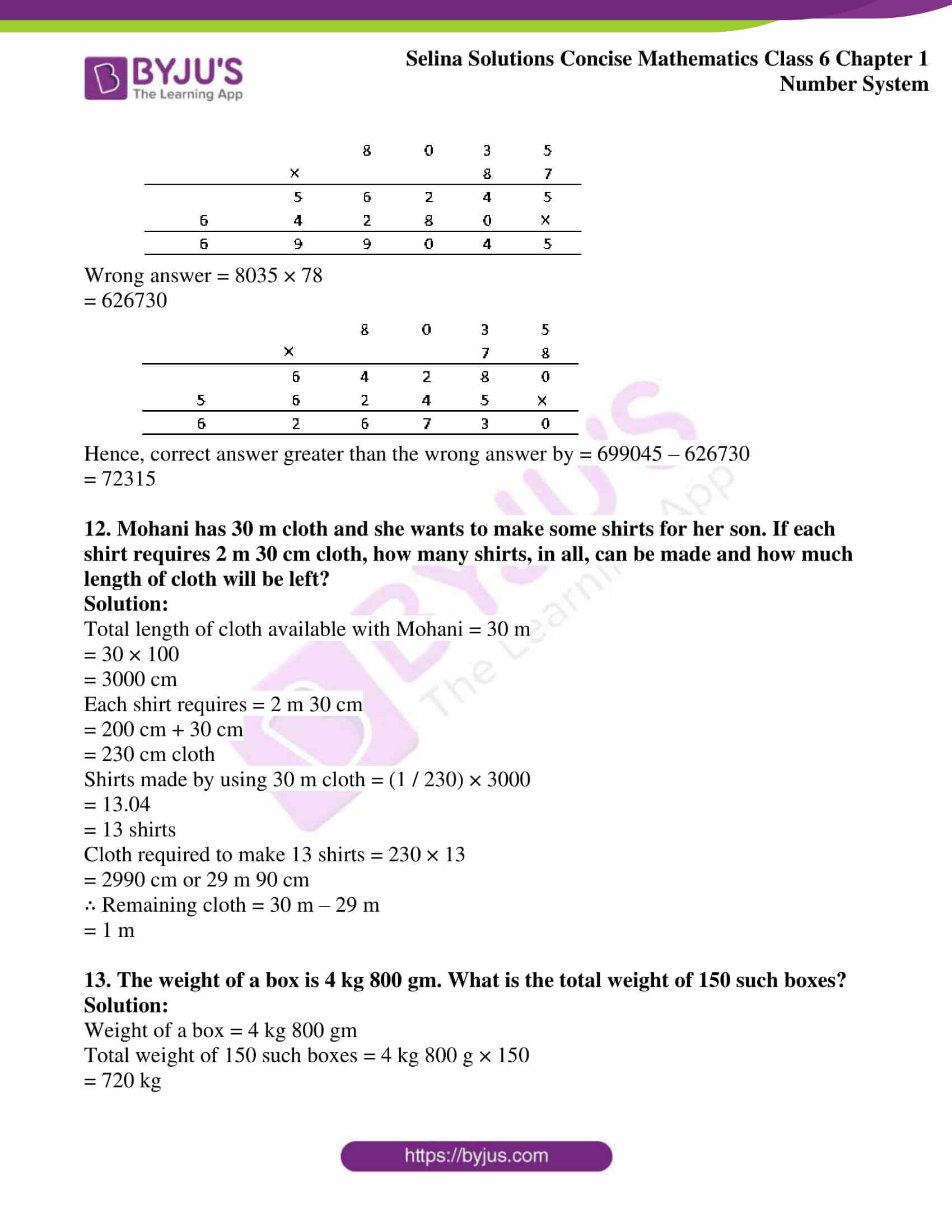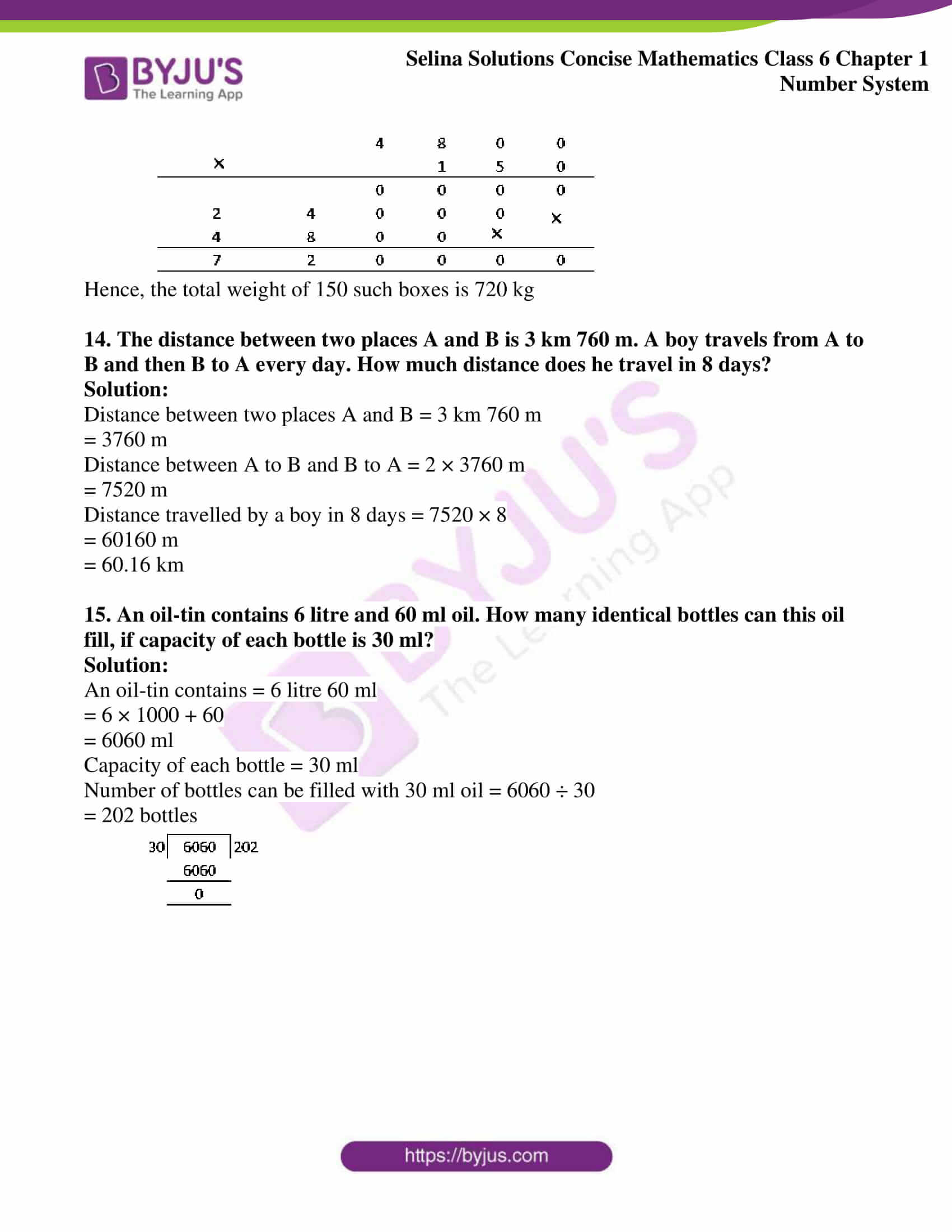### Exercise 1(a) Page no: 5

1. Which is greater?
(i) 537 or 98
(ii) 2428 or 529
(iii) 2, 59, 467 or 10, 35, 729

Solution:

(i) 537 or 98

Here 537 is a three digit number and 98 is a two digit number

∴537 > 98

(ii) 2428 or 529

Here 2428 is a four digit number and 529 is a three digit number

∴2428 > 529

(iii) 2, 59,467 or 10, 35,729

Here 10, 35,729 is a seven digit number and 2, 59,467 is a six digit number

∴10,35,729 > 2,59,467

2. Which is smaller?
(i) 428 or 437
(ii) 2497 or 2597
(iii) 3297 or 3596

Solution:

(i) 428 or 437

We observe that both the numbers are of three digit numbers

At the leftmost, both the number have same digit i.e. 4.

At the second place from left, the first number has 2 and the second number has 3

We know that,

2 < 3

∴ 428 is smaller than 437

(ii) 2497 or 2597

We observe that both the numbers are of four digit numbers.

At the leftmost, both the numbers have same digit i.e. 2

At the second place from the left, the first number has 4 and the second number has 5

We know that,

4 < 5

∴2497 is smaller than 2597

(iii) 3297 or 3596

We observe that both the number are of four digit numbers

At the leftmost, both the numbers have same digit i.e. 3

At the second place from the left, the first number has 2 and the second number has 5

We know that,

2 < 5

∴3297 is smaller than 3596

3.Which is greater?
(i) 45293 or 45427
(ii) 380362 or 381007
(iii) 63520 or 63250

Solution:

(i) 45293 or 45427

We observe that both the numbers are of 5 digits numbers

The digits are same at the leftmost and second place from left

But the digits at the third place from the left are different, the first number has 2 and the second number has 4

We know that,

2 < 4

Hence, 45427 is greater than 45293

(ii) 380362 or 381007

We observe that both the numbers are of 6 digits numbers

The digits are same at the leftmost and second place from left

But the digits at the third place from left are different, the first number has 0 and the second number has 1

We know that,

0 < 1

Hence, 381007 is greater than 380362

(iii) 63520 or 63250

We observe that both the numbers are of 5 digits numbers

The digits are same at the leftmost and second place from left

But the digits at the third place from left are different, the first number has 5 and the second number has 2

We know that,

2 < 5

Hence, 63520 is greater than 63250

4. By making a suitable chart, compare:
(i) 540276 and 369998
(ii) 6983245 and 6893254

Solution:

(i) 540276 and 369998

 5 4 0 2 7 6 3 6 9 9 9 8

Here, both the numbers have equal number of digits i.e. 6

At the leftmost, the first number has 5 and the second number has 3

Since 5 > 3

540276 is greater than 369998

(ii) 6983245 and 6893254

 6 9 8 3 2 4 5 6 8 9 3 2 5 4

Here, both the numbers have equal number of digits i.e. 7

At the leftmost, both the numbers have the same digit i.e. 6

At the second place from left, the first number has 9 and the second number has 8

Since, 9 > 8

6983245 is greater than 6893254

5.Compare the numbers written in the following table by writing them in ascending order:

 5 4 3 2 9 7 2 2 3 1 0 6 2 9 3 5 2 2 3 7 9 1 2 3 1 8 2 6 3 4 5 4 3 4 4 7 8 2

Solution:

The given numbers in the ascending order are

5223791< 5432972 < 23106293 < 23182634 < 54344782

 5 2 2 3 7 9 1 5 4 3 2 9 7 2 2 3 1 0 6 2 9 3 2 3 1 8 2 6 3 4 5 4 3 4 4 7 8 2

6.Use table form to compare the numbers in descending order:

5, 43,287; 54,82,900; 27,32,940; 43, 877; 78,396 and 4,999

Solution:

The given numbers in descending order are

5482900 > 2732940 > 543287 > 78396 > 43877 > 4999

 5 4 8 2 9 0 0 2 7 3 2 9 4 0 5 4 3 2 8 7 7 8 3 9 6 4 3 8 7 7 4 9 9 9

7. Find the smallest and the greatest numbers in each case given below:
(i) 983, 5754, 84 and 5942
(ii) 32849, 53628, 5499 and 54909.

Solution:

(i) 983, 5754, 84 and 5942

Since 84 has the least number of digits.

84 is the smallest number

5754 and 5942 have the maximum number of digits. Among these two 5942 is greater than 5754

Therefore 84 is the smallest number and 5942 is the greatest number

(ii)32849, 53628, 5499 and 54909.

Since 5499 has the least number of digits.

5499 is the smallest

54909 and 53628 have the maximum number of digits. Among these two 54909 is the greater than 53628

Therefore 5499 is the smallest number and 54909 is the greatest number

8.Form the greatest and the smallest 4 digit numbers using the given digits without repetition
(i) 3, 7, 2 and 5
(ii) 6, 1, 4 and 9
(iii) 7, 0, 4 and 2
(iv) 1, 8, 5 and 3
(v) 9, 6, 0 and 7

Solution:

(i) The given digits are 3, 7, 2 and 5

(a) 7532 is the greatest 4-digit number

(b) 2357 is the smallest 4-digit number

(ii) The given digits are 6, 1, 4 and 9

(a) 9641 is the greatest 4-digit number

(b) 1469 is the smallest 4-digit number

(iii) The given digits are 7, 0, 4 and 2

(a) 7420 is the greatest 4-digit number

(b) 2047 is the smallest 4-digit number

(iv)The given digits are 1, 8, 5, and 3

(a) 8531 is the greatest 4-digit number

(b) 1358 is the smallest 4-digit number

(v) The given digits are 9, 6, 0 and 7

(a) 9760 is the greatest 4-digit number

(b) 6079 is the smallest 4-digit number

9. Form the greatest and the smallest 3-digit numbers using any three different digits with the condition that digit 6 is always at the unit (one’s) place.

Solution:

The greatest and the smallest 3-digit numbers using any three different digits with the condition that 6 is at the unit (one’s) place is

The greatest 3-digit number = 986

The smallest 3-digit number = 106

10. Form the greatest and the smallest 4-digit numbers using any four different digits, with the condition that digit 5 is always at ten’s place.

Solution:

The greatest and the smallest 4-digit numbers using any four different digits, with the condition that digit 5 is always at ten’s place is

The greatest 4-digit number = 9857

The smallest 4-digit number = 1052

11. Fill in the blanks:

(i) The largest number of 5 digits is …………. and the smallest number of 6-digits is …………….

(ii) The difference between the smallest number of four digits and the largest number of three digits = ………….. – …………… = …………..

(iii) The sum (addition) of the smallest number of three digits and the largest number of two digits = ………… + …………… = …………

(iv) On adding one to the largest five-digit number, we get ………………. which is the smallest …………… digit number.

(v) On subtracting one from the smallest four-digit number, we get ………….. which is the …………. three digit number.

Solution:

(i) The largest number of 5 digits is 99999 and the smallest number of 6-digits is 100000

(ii) The difference between the smallest number of four digits and the largest number of three digits = 1000 – 999 = 1

(iii) The sum (addition) of the smallest number of three digits and the largest number of two digits = 100 + 99 = 199

(iv) On adding one to the largest five digit number, we get 100000 which is the smallest 6-digit number

(v) On subtracting one from the smallest four digit number, we get 999 which is the greatest three digit number.

12. Form the largest number with the digits 2, 3, 5, 9, 6 and 0 without repetition of any digit.

Solution:

The largest number with the digits 2, 3, 5, 9, 6 and 0 without repetition of any digit is 965320

13. Write the smallest and the greatest numbers of 4 digits without repetition of any digit.

Solution:

The smallest and the greatest numbers of 4 digits without repetition of any digit are as follows

Smallest 4-digits number = 1023

Greatest 4-digits number = 9876

14. Find the greatest and the smallest five-digit numbers with 8 in hundred’s place and with all the digits different.

Solution:

The greatest and the smallest five-digit numbers with 8 in hundred’s place is

Greatest five-digits number = 97865

Smallest five-digits number = 10823

15. Find the sum of the largest and the smallest four-digit numbers.

Solution:

The largest four-digits number = 9999

The smallest four-digits number = 1000

Sum of the largest and the smallest four digits numbers = 9999 + 1000

= 10999

## Exercise 1(b) page no: 10

1. Population of a city was 354976 in the year 2014. In the year 2015, it increased by 68438. What was the population of the city at the end of the year 2015?

Solution:

Population of a city in the year 2014 = 354976

Population increased in the year 2015 = 68438

Total population at the end of the year 2015 = 354976 + 68438 = 423414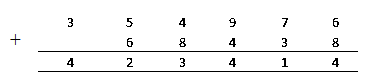2. A = 743000 and B = 800100. Which is greater A or B? And by how much?

Solution:

Given

A = 743000

B = 800100

B is greater than A i.e. (B > A)

Since both the numbers have 6-digits numbers

At the leftmost, the first number has 7 and the second number has 8

We know that,

8 > 7

Hence, 800100 > 743000 i.e B > A

B is greater than A by = 800100 – 743000

= 57100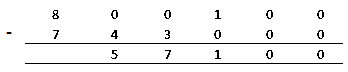3. A notebook has 56 pages. How many pages will 5326 such note-books have?

Solution:

Given

Number of pages in a note-book = 56 pages

Number of pages in 5326 note-books = 5326 × 56

= 298256 pages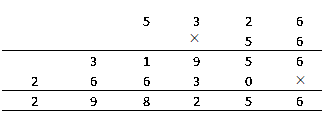4. A person has 75000 sheets of paper. If each sheet makes 8 pages of a note book, how many note-books of 200 pages can be made using the above sheets?

Solution:

Given

Number of sheets of paper available = 75000

Number of pages obtained from one sheet of paper = 8

Total number of pages obtained = 8 × 75000

= 600000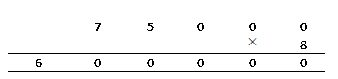We know that,

Number of notebooks made = (Total number of pages obtained) / (Number of pages contained by notebook)

= (600000) / (200)

= 3000

5. Add 176209; 450923; and 4483947

Solution: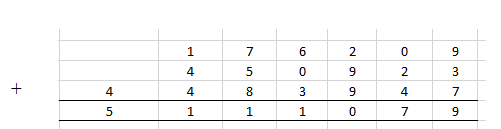Sum of 176209 + 450923 + 4483947 = 5111079

Therefore adding these values we get = 5111079

6. A cricket player has so far scored 7849 runs in test matches. He wishes to complete 10000 runs, how many more runs does he need?

Solution:

Given

A cricket player scored = 7849 runs

Total score he wish to complete = 10000

Runs required = 10000 – 7849 = 2151 runs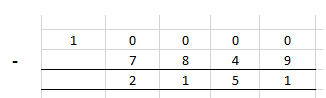Hence, a cricket player needs 2151 more runs to complete 10000 runs

7. In an election two candidates A and B are the only contestants. If candidate A scored 932567 votes and candidate B scored 900235 votes, by how much margin did A win or lose the election?

Solution:

Votes scored by candidate A = 932567

Votes scored by candidate B = 900235

Since votes scored by candidate A is more than B. Hence, candidate A win the election

Candidate A win the election by margin = 932567 – 900235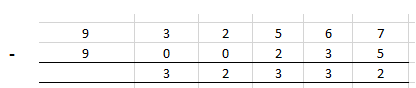8. Find the difference between the largest and the smallest five digits numbers that can be formed using the digits 5, 1, 6, 3 and 2 without repeating any digit.

Solution:

The given digits are = 5, 1, 6, 3 and 2

Using these digits 5, 1, 6, 3 and 2, the largest 5-digit number that can be formed = 65321

Using these digits 5, 1, 6, 3 and 2 the smallest 5-digit number that can be formed = 12356

Difference between 65321 and 12356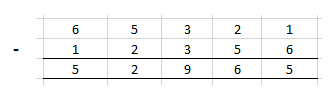= 65321 – 12356

= 52965

9. A machine manufactures 5782 screws every day. How many screws will it manufacture in the month of April?

Solution:

Number of screws manufactured in a day = 5782

Number of days in April = 30 days

Number of screws manufactured in April = 5782 × 30

= 173460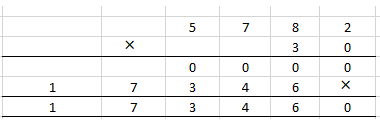Hence, a machine manufactures 173460 screws in the month of April

10. A man has ₹ 157184 with him. He placed an order for purchasing 80 articles at ₹ 125 each. How much money will remain with him after the purchase?

Solution:

A man has = ₹ 157184

Number of articles purchased by him = 80

Cost of one article = ₹ 125

Cost of 80 articles will be = 80 × 125

= ₹ 10000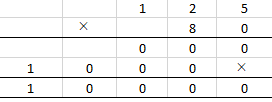Remaining money with him after the purchase = Money in hand – Cost of 80 articles

= 157184 – 10000

= 147184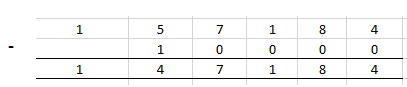11. A student multiplied 8035 by 87 instead of multiplying by 78. By how much was his answer greater than or less than the correct answer?

Solution:

Correct answer = 8035 × 87

= 699045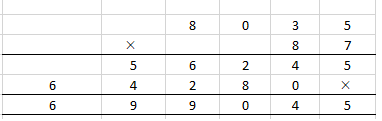Wrong answer = 8035 × 78

= 626730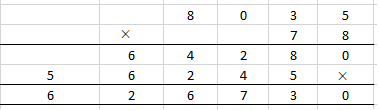Hence, correct answer greater than the wrong answer by = 699045 – 626730

= 72315

12. Mohani has 30 m cloth and she wants to make some shirts for her son. If each shirt requires 2 m 30 cm cloth, how many shirts, in all, can be made and how much length of cloth will be left?

Solution:

Total length of cloth available with Mohani = 30 m

= 30 × 100

= 3000 cm

Each shirt requires = 2 m 30 cm

= 200 cm + 30 cm

= 230 cm cloth

Shirts made by using 30 m cloth = (1 / 230) × 3000

= 13.04

= 13 shirts

Cloth required to make 13 shirts = 230 × 13

= 2990 cm or 29 m 90 cm

∴ Remaining cloth = 30 m – 29 m

= 1 m

13. The weight of a box is 4 kg 800 gm. What is the total weight of 150 such boxes?

Solution:

Weight of a box = 4 kg 800 gm

Total weight of 150 such boxes = 4 kg 800 g × 150

= 720 kg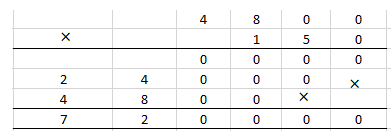Hence, the total weight of 150 such boxes is 720 kg

14. The distance between two places A and B is 3 km 760 m. A boy travels from A to B and then B to A every day. How much distance does he travel in 8 days?

Solution:

Distance between two places A and B = 3 km 760 m

= 3760 m

Distance between A to B and B to A = 2 × 3760 m

= 7520 m

Distance travelled by a boy in 8 days = 7520 × 8

= 60160 m

= 60.16 km

15. An oil-tin contains 6 litre and 60 ml oil. How many identical bottles can this oil fill, if capacity of each bottle is 30 ml?

Solution:

An oil-tin contains = 6 litre 60 ml

= 6 × 1000 + 60

= 6060 ml

Capacity of each bottle = 30 ml

Number of bottles can be filled with 30 ml oil = 6060 ÷ 30

= 202 bottles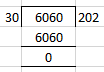## Exercises of Selina Solutions Concise Mathematics Class 6 Chapter 1: Number System

Exercise 1(A) Solutions

Exercise 1(B) Solutions